Ex 13.5 (Optional)

Chapter 13 Class 10 Surface Areas and Volumes
Serial order wise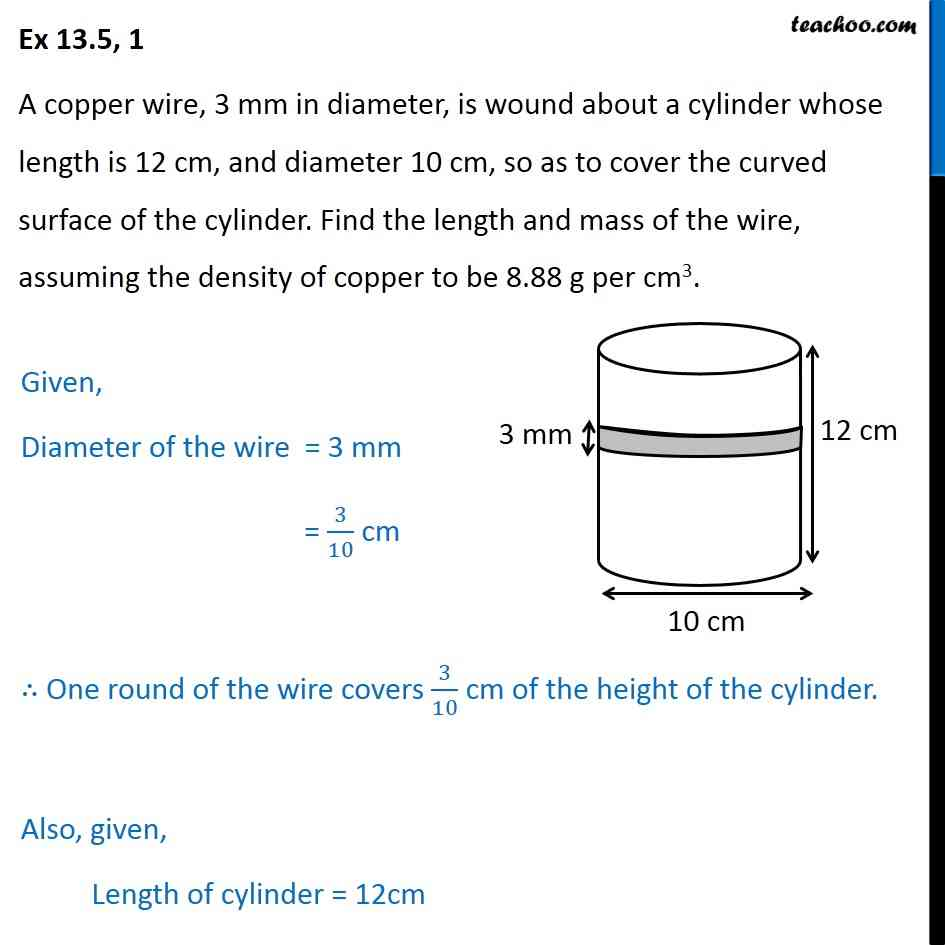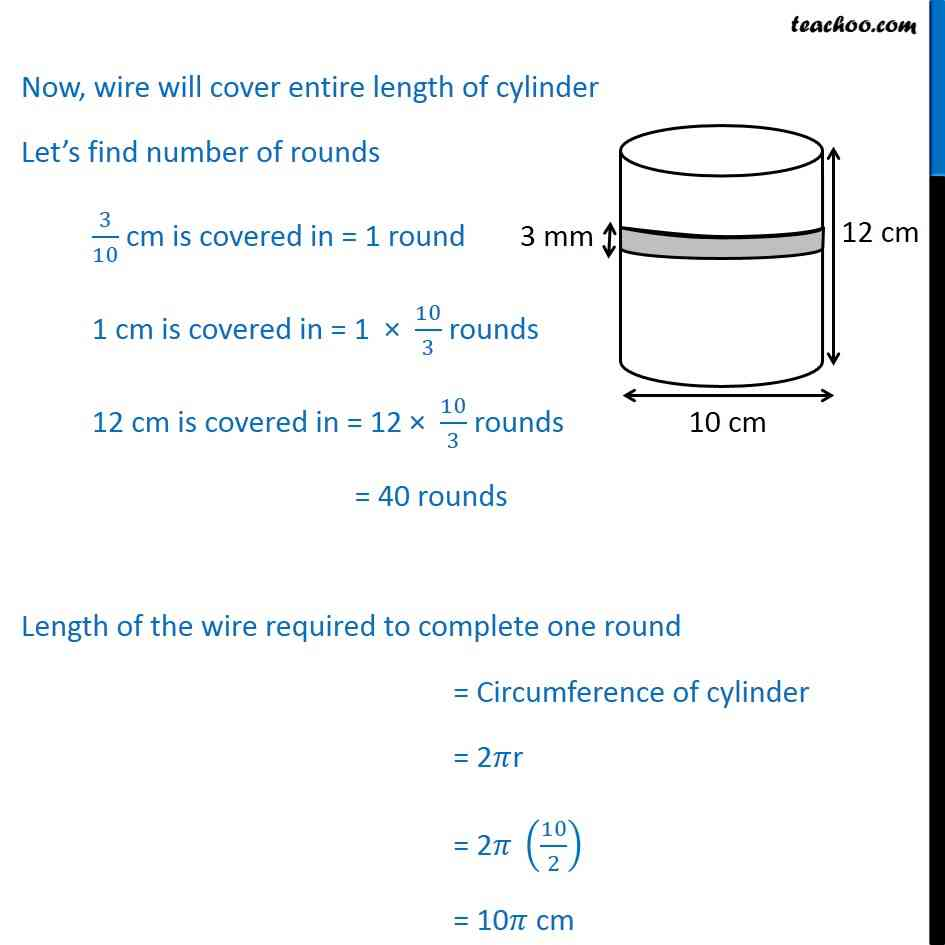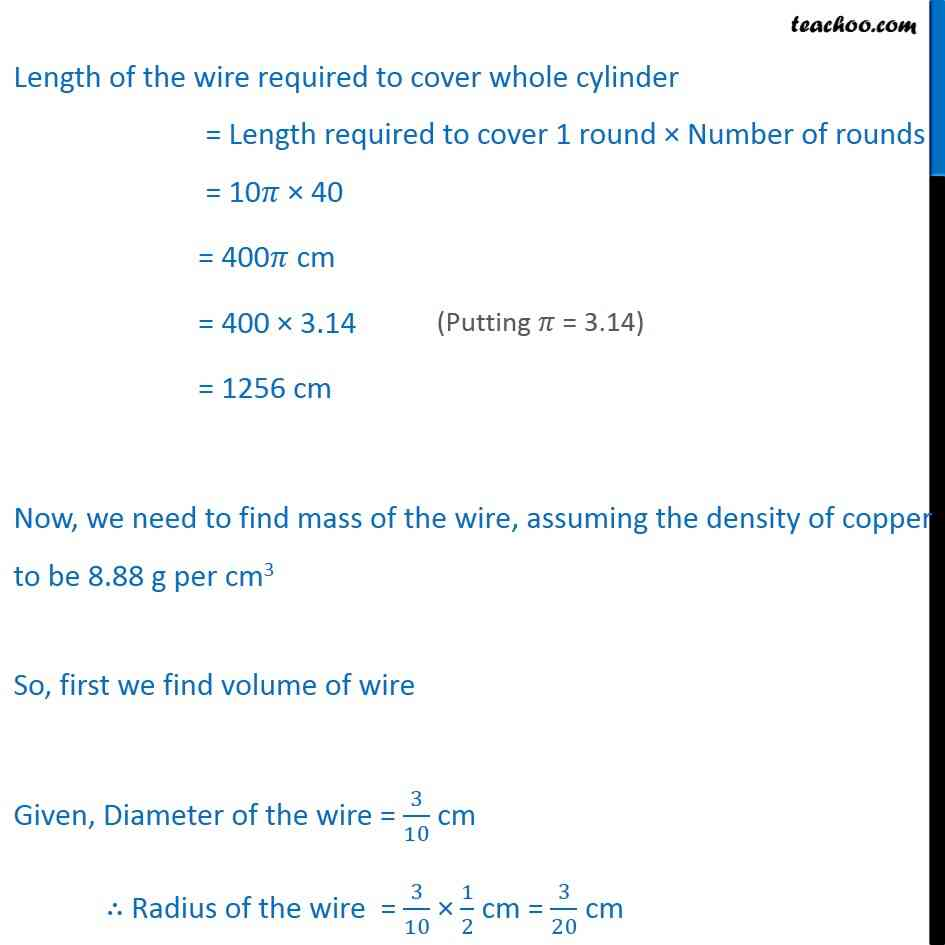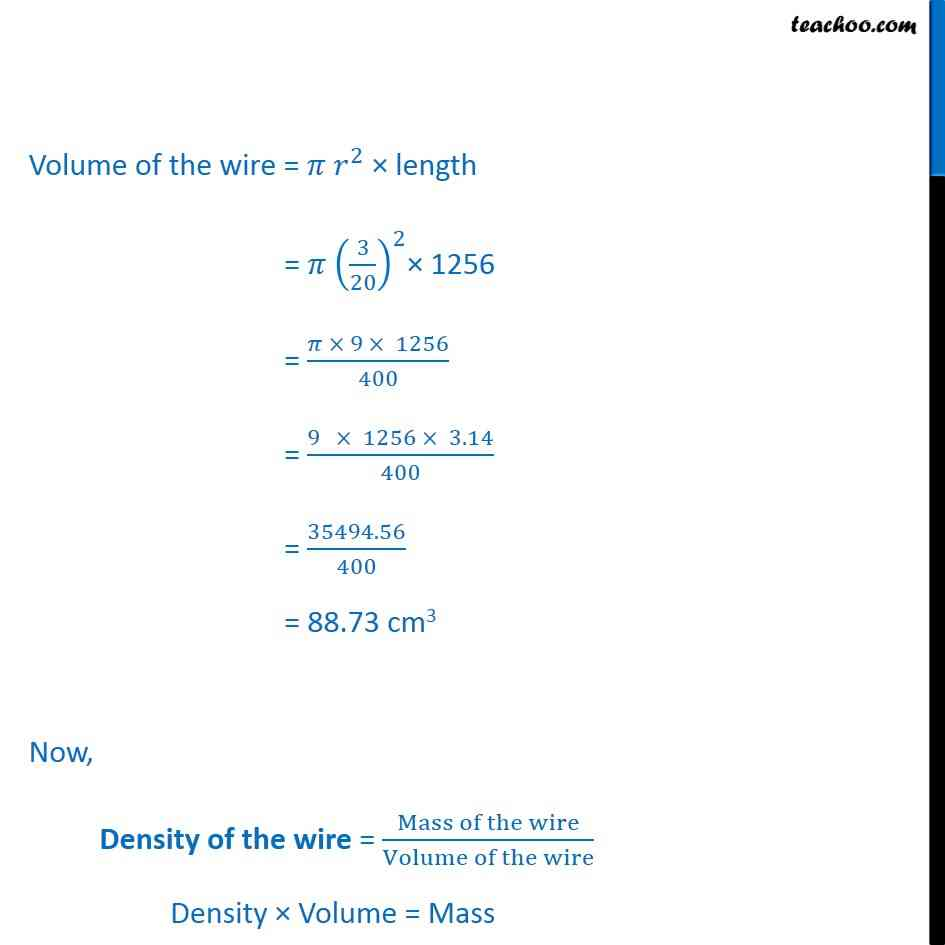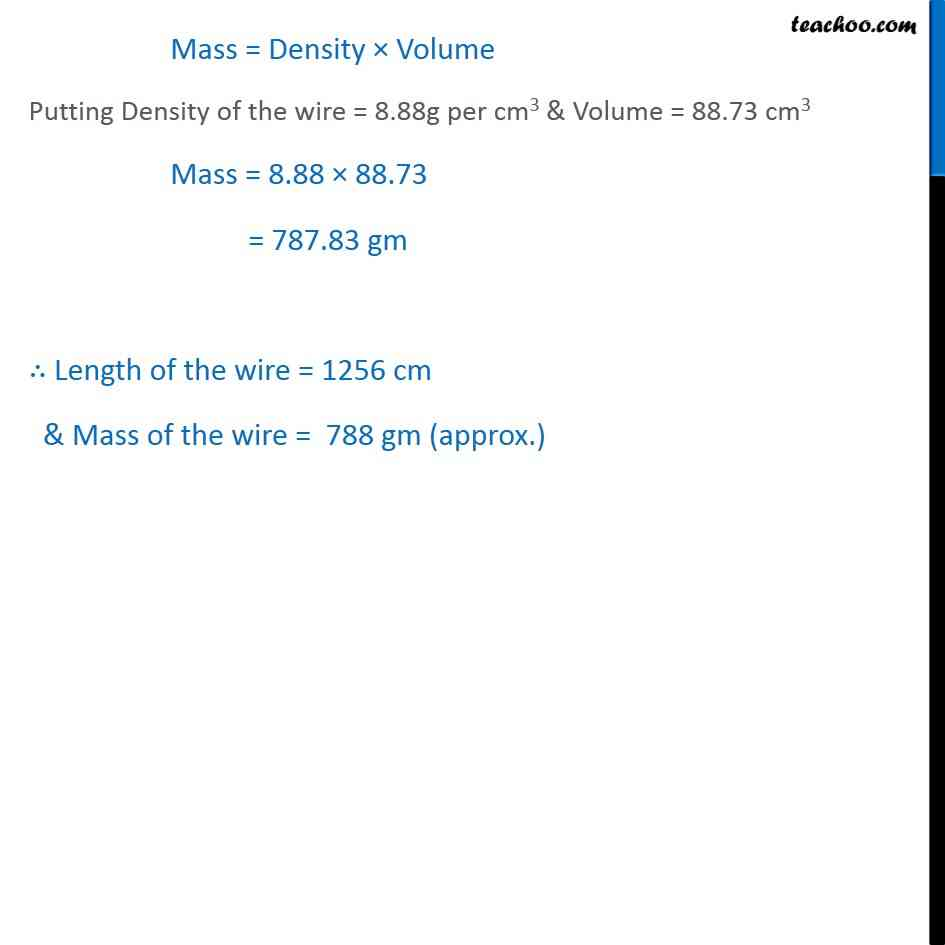Get live Maths 1-on-1 Classs - Class 6 to 12

### Transcript

Ex 13.5, 1 A copper wire, 3 mm in diameter, is wound about a cylinder whose length is 12 cm, and diameter 10 cm, so as to cover the curved surface of the cylinder. Find the length and mass of the wire, assuming the density of copper to be 8.88 g per cm3. Given, Diameter of the wire = 3 mm = 3/10 cm One round of the wire covers 3/10 cm of the height of the cylinder. Also, given, Length of cylinder = 12cm Now, wire will cover entire length of cylinder Let s find number of rounds 3/10 cm is covered in = 1 round 1 cm is covered in = 1 10/3 rounds 12 cm is covered in = 12 10/3 rounds = 40 rounds Length of the wire required to complete one round = Circumference of cylinder = 2 r = 2 (10/2) = 10 cm Length of the wire required to cover whole cylinder = Length required to cover 1 round Number of rounds = 10 40 = 400 cm = 400 3.14 = 1256 cm Now, we need to find mass of the wire, assuming the density of copper to be 8.88 g per cm3 So, first we find volume of wire Given, Diameter of the wire = 3/10 cm Radius of the wire = 3/10 1/2 cm = 3/20 cm Volume of the wire = ^2 length = (3/20)^2 1256 = ( 9 1256)/400 = (9 1256 3.14)/400 = 35494.56/400 = 88.73 cm3 Now, Density of the wire = (Mass of the wire)/(Volume of the wire) Density Volume = Mass Mass = Density Volume Putting Density of the wire = 8.88g per cm3 & Volume = 88.73 cm3 Mass = 8.88 88.73 = 787.83 gm Length of the wire = 1256 cm & Mass of the wire = 788 gm (approx.)